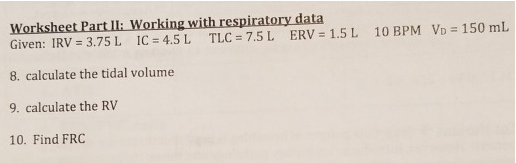# Problem: Given: IRV = 3.75 L     IC = 4.5 L    TLC =7.5 L    ERV = 1.5 L    10 BPM VD = 150 mL  8. Calculate the tidal volume 9. Calculate the RV 10. Find FRC

###### FREE Expert Solution

8. Tidal volume (TV) refers to the usual and normal amount of air volume difference between inspired and expired gases without any extra effort. Thus, the tidal volume can be calculated as below:

IC = TV + IRV

Inserting the values given, we get:

4.5 = TV + 3.75

Thus, TV = 4.5 - 3.75 OR 0.75 L OR 750 mL###### Problem Details

Given: IRV = 3.75 L     IC = 4.5 L    TLC =7.5 L    ERV = 1.5 L    10 BPM VD = 150 mL

8. Calculate the tidal volume

9. Calculate the RV

10. Find FRC